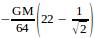#### Thank you for registering.

One of our academic counsellors will contact you within 1 working day.

Click to Chat

1800-5470-145

+91 7353221155

CART 0

• 0
MY CART (5)

Use Coupon: CART20 and get 20% off on all online Study Material

ITEM
DETAILS
MRP
DISCOUNT
FINAL PRICE
Total Price: Rs.

There are no items in this cart.
Continue Shopping

# A solid sphere of uniform density and radius 4 units is located with centre at origin.Two spheres of equal radii 1 unit, with centres at A(-2,0,0) and B (2,0,0) are taken out of the solidleaving behind spherical cavities.Then: the gravitational force due to this at origin is zero. the gravitational force at B is zero the gravitational potential is same at all points of the circle y2+ z2= 36 the gravitational potential at all points on the circle y2 + z2= 4

6 years ago
p { margin-bottom: 0.25cm; line-height: 120%; }p { margin-bottom: 0.25cm; line-height: 120%; }

Dear student, please see the picture below.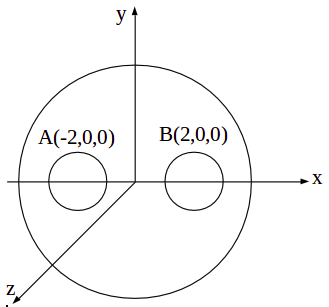p { margin-bottom: 0.25cm; line-height: 120%; }

Let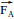= gravitational field due to sphere A (if it were present at that position)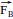= gravitational field due to sphere B (if it were present at that position)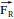= gravitational field due to remaining portion after the cavities are made.

Then from superposition principle, we can see that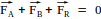, as the force due to entire sphere is zero at center.

Now since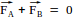due to symmetry.

Hence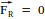, hence option option (i) is correct

Now at B,

Field due to entire sphere is given by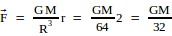,

whereas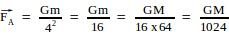, where m = mass of sphere A = M/64

and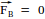From superposition principle, we have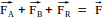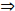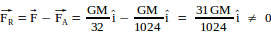,

Hence option (ii) is not correct.

Regarding Potential at a point on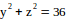, we can see that the radius of the circle is 6 units, however, all the points on it are symmetrically located from remaining sphere. Hence potential must be same at every point on this circle. Same logic holds for the circle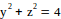also, though this circle lies inside the remaining sphere.

Hence options (iii) and (iv) are also correct.

Hence options (i), (iii) and (iv) are correct.

Note, we can use superposition principle to calculate the potential at these points,

In option (iii) it will be equal to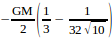In option (iv) it will be equal to